# Polynomial ring over integrally closed subring is integrally closed in polynomial ring

## Statement

Suppose$R$ is an integrally closed subring of a commutative unital ring$S$. Then, the polynomial ring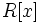$R[x]$ is an integrally closed subring of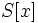$S[x]$.

## Proof

Given: A ring$S$, an integrally closed subring$R$.

To prove:$R[x]$ is an integrally closed subring of$S[x]$.

Proof: Suppose$f \in S[x]$ satisfies a monic polynomial over$R[x]$:$f^n + g_{n-1}f^{n-1} + g_{n-2}f^{n-2} + \dots + g_0 = 0$.

Here,$g_i \in R[x]$ for all math>i[/itex]. Write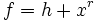$f = h + x^r$, for$r$ greater than the maximum of the degrees of the$g_i$. Note that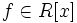$f \in R[x]$ if and only if$h \in R[x]$, so it suffices to show that$h \in R[x]$.$h$ satisfies the monic polynomial: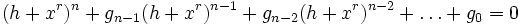$(h + x^r)^n + g_{n-1}(h + x^r)^{n-1} + g_{n-2}(h + x^r)^{n-2} + \dots + g_0 = 0$.

The constant term of this, viewed as a polynomial in$h$, is:$(x^r)^n + g_{n-1}(x^r)^{n-1} + \dots + g_0$.

Since all the$g_i$ are in$R[x]$, this whole constant term is an element of$R[x]$.

Fill this in later